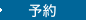•Essentials of Modern Business Statistics with Microsoft Excel (Mindtap Course List) （8TH）

• ただいまウェブストアではご注文を受け付けておりません。 ⇒古書を探す
• 製本 Hardcover:ハードカバー版
• 言語 ENG,ENG
• 商品コード 9780357131626
• DDC分類 310

Full Description

Develop a strong conceptual understanding of statistics as ESSENTIALS OF MODERN BUSINESS STATISTICS WITH MICROSOFT (R) EXCEL (R), 8E balances real-world applications with an integrated focus on the latest version of Microsoft (R) Excel (R). This best-selling, essentials edition clearly develops each statistical technique in an application setting. You learn to master statistical methodology with an easy-to-follow presentation of a statistical procedure followed by a discussion of how to use Excel (R) 2019 to perform the procedure. Step-by-step instructions and screen captures ensure understanding.More than 140 new business examples, proven methods, and application exercises show how statistics provide insights into today's business decisions and problems. A unique problem-scenario approach and new case problems demonstrate how to apply statistical methods to practical business situations. MindTap digital resources provide tools to help you master Excel (R), Excel (R) Online, and R as well as gain an understanding of business statistics.

Contents

1. Data and Statistics.2. Descriptive Statistics: Tabular and Graphical Displays.3. Descriptive Statistics: Numerical Measures.4. Introduction to Probability.5. Discrete Probability Distributions.6. Continuous Probability Distributions.7. Sampling and Sampling Distributions.8. Interval Estimation.9. Hypothesis Tests.10. Inferences About Means and Proportions with Two Populations.11. Inferences About Population Variances.12. Test of Goodness of Fit, Independence, and Multiple Proportions.13. Experimental Design and Analysis of Variance.14. Simple Linear Regression.15. Multiple Regression.Appendix A: References and Bibliography.Appendix B: Tables.Appendix C: Summation Notation.Appendix D: Self-Test Solutions and Answers to Even-Numbered Exercises (online).Appendix E: Microsoft Excel 2016 and Tools for Statistical Analysis.